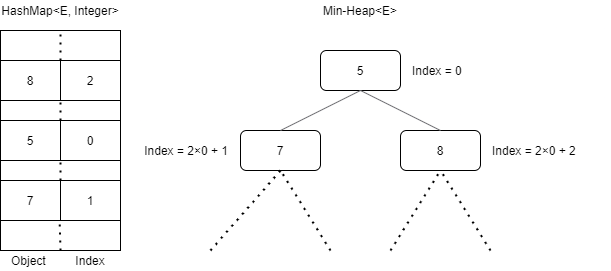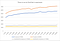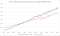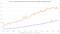# Solving K-Max problem combining PriorityQueue and HashMap data structure

Our problem here, is to find the k-max values in a very large dataset of n elements without duplicates such as k << n.

In this article I will explain how I implemented a modified version of the java PriorityQueue to solve the given problem.Illustration of combining PriorityQueue and HashMap for an Integer object.

The first idea was to solve the problem using a TreeSet. It has many advantages such as ordered sorting and it does not allow duplicates. However according to the Java Doc inserting or checking if an element is already inside has a complexity of O(log n). Therefore we understand that for very large set of data we are losing a lot of time simply trying to insert elements which could already be inside.

In order to solve this problem, I took the build-in implementation of the PriorityQueue and modified some methods. It was not possible to extend the class PriorityQueue<E> to achieve my goal because some deep modification in privates methods were necessary.

The principle is to keep in the HashTable, a record of the index of every element in the queue array. Therefore when checking if an element is in the table we achieve it in O(1) instead of O(log n). This allow us to save a lot of time simply by increasing a bit the memory used by the algorithm.

The first modification is to add a transient HashMap<E,Integer> field in our class (E is our Object, could be any type). Because we already know the maximum number the HashMap will contain, which is k. We can change the initial size setting to optimize it. The default load factor for an HashMap is 0.75, I advise to let this value and put the hashMapCapacity to 134% of k, to have a load factor corresponding to our maximum number of value.

The following methods have been modified in order to keep track of the positions of all elements when we are moving them:

• siftUpComparable/siftUpUsingComparator
• siftDownComparable/siftDownUsingComparator

We simply update the integer value corresponding to the object in the HashMap to remember where it is.

Another important improvement in comparison to the default implementation is that we will not resize the default array in our process because we already know the maximum number of element we will hold before starting it.

When the implementation is finished, we can use it as a normal collection. In this implementation we do not need to call the contains(E) methods because it is automatically checked in the add method before inserting.

Using this code, and the one previously demonstrated using the TreeSet structure, we can compare the performances.X-axis represent the number k-maximum values we want to keep and Y-axis the time to finish the algorithm in milliseconds.

In order to draw this chart, I generate for k from 500 to 9500 by 500 a set of random data (Random integer from 0 to n). Then I run the TreeSet algorithm and the ModifiedPriorityQueue algorithm on the same data and measure the time for each of them. Finally using org.apache.poi I exported the data to an excel document.

As I said in the introduction of this article, this is efficient for k << n, in fact when k starts to be too big, the time increases significantly.X-axis represent the number k-maximum values we want to keep and Y-axis the time to finish the algorithm in milliseconds. (Random data values from 0 to 100M)

In order to be able to compare the performance on larger value of k, we need to reduce the data set. Here n = 100M elements, and k varies from 1 000 to 50 000. We noticed that the ModifiedPriorityQueue is faster than the TreeSet for k < ~22 500. However after this point, the TreeSet seems to better manage the problem. This can be understood because the ModifiedPriorityQueue has been designed to be the most efficient as possible to avoid duplicated. So with a large set of values, we lose time inserting and deleting, and in this case a TreeSet is faster for bigger dataset.

In order to have a better understanding of the performance, I decided to run more test changing the variety of values in data samples.Data reduced to values between 0 and 600 000.

In this new chart, where the data varieties are reduced to an interval from 0 to 600 000, we see that the ModifiedPriorityQueue is way more efficient even for larger k.

# Conclusion

I implemented this idea to solve a problem on a large scale of data I had. I found this solution suitable for my needs. As it is visible on the charts, depending on the data, it is sometimes better to use build-in data structure. The source-code is available on my GitHub.

French student in engineering school.

## More from axel7083

French student in engineering school.